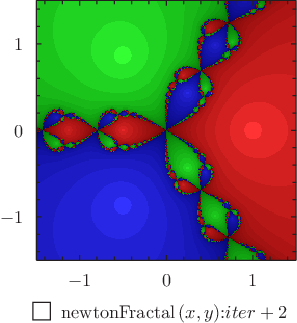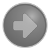# Pyxplot

## Examples - Newton fractalDownload this example:

Example plot - using the colormap plot style to draw the Newton fractal

The Newton fractal for the polynomial z**3-1, plotted using the colormap plot style. In addition to coloring points red, green or blue depending upon which root of the polynomial the Newton-Raphson iteration converges to, we also assign points different brightnesses depending upon how long the iteration takes to converge.

### Script

```set numerics complex
set unit angle nodimensionless

root1 = exp(i*unit(  0*deg))
root2 = exp(i*unit(120*deg))
root3 = exp(i*unit(240*deg))

tolerance = 1e-2

subroutine newtonFractal(x,y)
{
global iter
z = x+i*y
iter = 0
while (1)
{
z = z - (z**3-1)/(3*z**2)
if abs(z-root1)
```